# Dask – How to handle large dataframes in python using parallel computingDask provides efficient parallelization for data analytics in python. Dask Dataframes allows you to work with large datasets for both data manipulation and building ML models with only minimal code changes. It is open source and works well with python libraries like NumPy, scikit-learn, etc. Let’s understand how to use Dask with hands-on examples.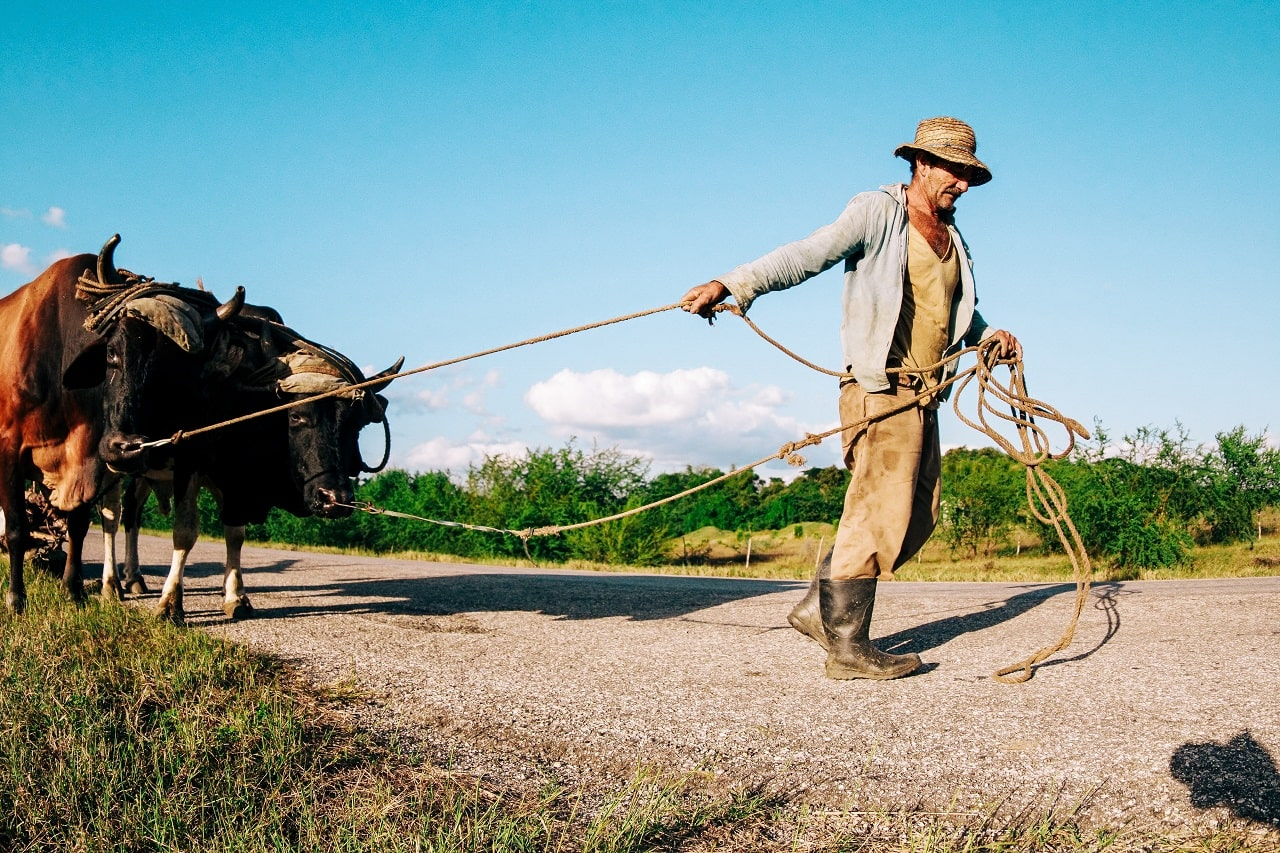Dask – How to handle large data in python using parallel computing

## Contents

Get FREE 30 DAY ACCESS  to Structured learning path for ML in Python (Beginner to Pro) –  Start as an absolute beginner and graduate over 15 courses to solve Industrial Projects like a real Data Scientist. Includes query support over chat.

## Why do you need Dask?

Python packages like numpy, pandas, sklearn, seaborn etc. makes the data manipulation and ML tasks very convenient. For most data analysis tasks, the python pandas package is good enough. You can do all sorts of data manipulation and is compatible for building ML models.

But, as your data gets bigger, bigger than what you can fit in the RAM, pandas won’t be sufficient.

This is a very common problem.

You may use Spark or Hadoop to solve this. But, these are not python environments. This stops you from using numpy, sklearn, pandas, tensorflow, and all the commonly used Python libraries for ML.

Is there a solution for this?

Yes! This is where Dask comes in.

Dask is a open-source library that provides advanced parallelization for analytics, especially when you are working with large data.

It is built to help you improve code performance and scale-up without having to re-write your entire code. The good thing is, you can use all your favorite python libraries as Dask is built in coordination with numpy, scikit-learn, scikit-image, pandas, xgboost, RAPIDS and others.

That means you can now use Dask to not only speed up computations on datasets using parallel processing, but also build ML models using scikit-learn, XGBoost on much larger datasets.

You can use it to scale your python code for data analysis. If you think, this sounds a bit complicated to implement, just read on.

So, What is Parallel Processing?

Parallel processing refers to executing multiple tasks at the same time, using multiple processors in the same machine.

Generally, the code is executed in sequence, one task at a time. But, let’s suppose, you have a complex code that takes a long time to run, but mostly the code logics are independent, that is, no data or logic dependency on each other. This is the case for most matrix operations.

So, instead of waiting for the previous task to complete, we compute multiple steps simultaneously at the same time. This lets you take advantage of the available processing power, which is the case in most modern computers, thereby reducing the total time taken.

Dask is designed to do this efficiently on datasets with minimal learning curve. Let’s see how.

## How to implement Parallel Processing with Dask

A very simple way is to use the dask.delayed decorator to implement parallel processing. Let me explain it through an example.

Consider the below code snippet.

from time import sleep

def apply_discount(x):
sleep(1)
x=x-0.2*x
return x

def get_total(a,b):
sleep(1)
return a+b

def get_total_price(x,y):
sleep(1)
a=apply_discount(x)
b=apply_discount(y)
get_total(a,b)


Given a number, the above code simply applies a 20 percent discount on price and then add them. I’ve inserted a sleep function explicitly so both the functions take 1sec to run. This is a small code that will run quickly, but I have chosen this to demonstrate for beginners.

%%time
# This takes three seconds to run because we call each
# function sequentially, one after the other

x = apply_discount(100)
y = apply_discount(200)
z = get_total_price(x,y)


CPU times: user 859 µs, sys: 202 µs, total: 1.06 ms
Wall time: 6.01 s

I have recorded the time taken for this execution using %%time as shown. You can observe that time taken is 6.01 seconds, when it is executed sequentially. Now, let’s see how to use dask.delayed to reduce this time.

# Import dask and and dask.delayed


Now, you can transform the functions apply_discount() and get_total_price(). You can use delayed() function to wrap the function calls that you want to turn into tasks.

# Wrapping the function calls using dask.delayed
x = delayed(apply_discount)(100)
y = delayed(apply_discount)(200)
z = delayed(get_total_price)(x, y)


Get FREE 30 DAY ACCESS  to Structured learning path for ML in Python (Beginner to Pro) –  Start as an absolute beginner and graduate over 15 courses to solve Industrial Projects like a real Data Scientist. Includes query support over chat.

It creates a delayed object, that keeps track of all the functions to call and the arguments to pass to it. Basically, it builds a task graph that explains the entire computation. It helps to spot opportunities for parallel execution.

So, the z object created in the above code is a delayed object OR “lazy object” which has all information for executing the logic. You can see the optimal task graph created by dask by calling the visualize() function.

z.visualize()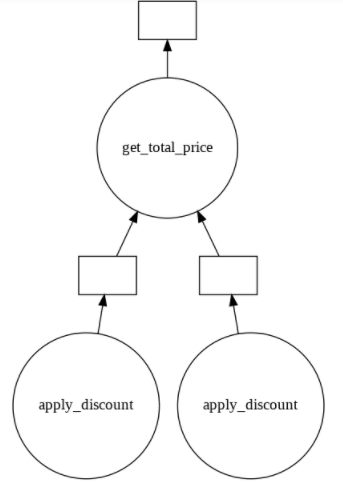Clearly from the above image, you can see there are two instances of apply_discount() function called in parallel. This is an opportunity to save time and processing power by executing them simultaneously.

Up until now, only the logic to compute the output, that is the task graph is computed. To actually execute it, let’s call the compute() method of z.

%%time
z.compute()


CPU times: user 6.33 ms, sys: 1.35 ms, total: 7.68 ms
Wall time: 5.01 s

Though it’s just 1 sec, the total time taken has reduced. This is the basic concept of parallel computing. Dask makes it very convenient.

Let’s now look at more useful examples.

## Example: Parallelizing a for loop with Dask

In the previous section, you understood how dask.delayed works. Now, let’s see how to do parallel computing in a for-loop.

Consider the below code.

You have a for-loop, where for each element a series of functions is called.

In this case, there is a lot of opportunity for parallel computing. Again, we wrap the function calls with delayed(), to get the parallel computing task graph.

# Functions to perform mathematics operations
def square(x):
return x*x

def double(x):
return x*2

return x + y

# For loop that calls the above functions for each data
output = []
for i in range(6):
a = delayed(square)(i)
b = delayed(double)(i)
output.append(c)

# Visualizing the task graph for the problem
total.visualize()


For this case, the total variable is the lazy object. Let’s visualize the task graph using total.visualize().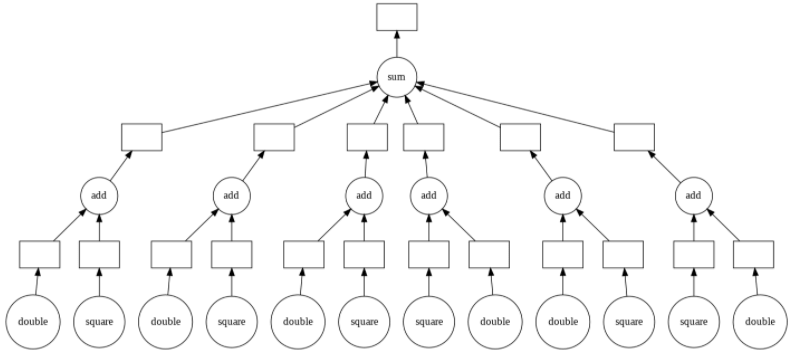You can see from above that as problems get more complex, so here, parallel computing becomes more useful and necessary.

Now, wrapping every function call inside delayed() becomes laborious. But then, the delayed function is actually a Decorator. So, you can just add the @delayed decorator before the function definitions as shown below. This reduces the number of code changes.

# Using delayed as a decorator to achieve parallel computing.

@delayed
def square(x):
return x*x

@delayed
def double(x):
return x*2

@delayed
return x + y

# No change has to be done in function calls
output = []
for i in range(6):
a = square(i)
b = double(i)
output.append(c)

total.visualize()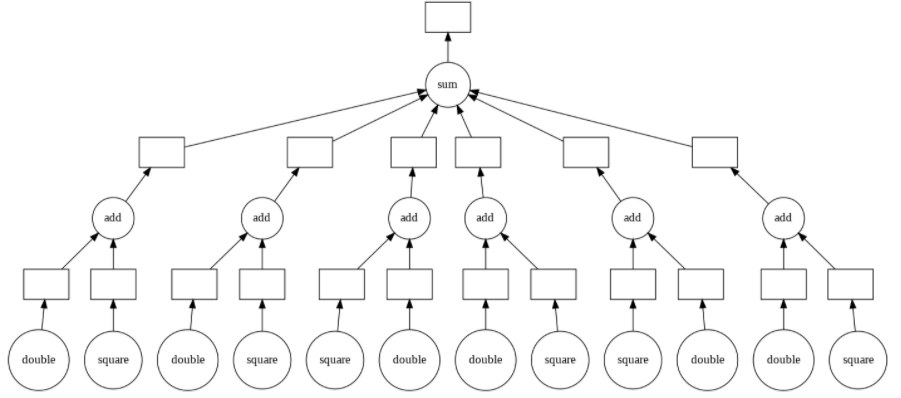As expected, you get the same output.

So you can use delayed as a decorator as is and it will parallelize a for-loop as well. Isn’t that awesome?

## Dask DataFrames – How to use them?

You saw how Dask helps to overcome the problem of long execution and training time. Another important problem we discussed was the larger-than-memory datasets.

The commonly used library for working with datasets is Pandas. But, many real-life ML problems have datasets that are larger than your RAM memory!

In these cases, Dask Dataframes is useful. You can simply import the dataset as dask.dataframe instead, which you can later convert to a pandas dataframe after necessary wrangling/calculations are done.

## How is dask.dataframe different from pandas.dataframe?

A Dask DataFrame is a large parallel DataFrame composed of many smaller Pandas DataFrames, split along the index. One Dask DataFrame is comprised of many in-memory pandas DataFrames separated along with the index.

These Pandas DataFrames may live on disk for larger-than-memory computing on a single machine, or on many different machines in a cluster. One Dask DataFrame operation triggers many operations on the constituent Pandas DataFrames.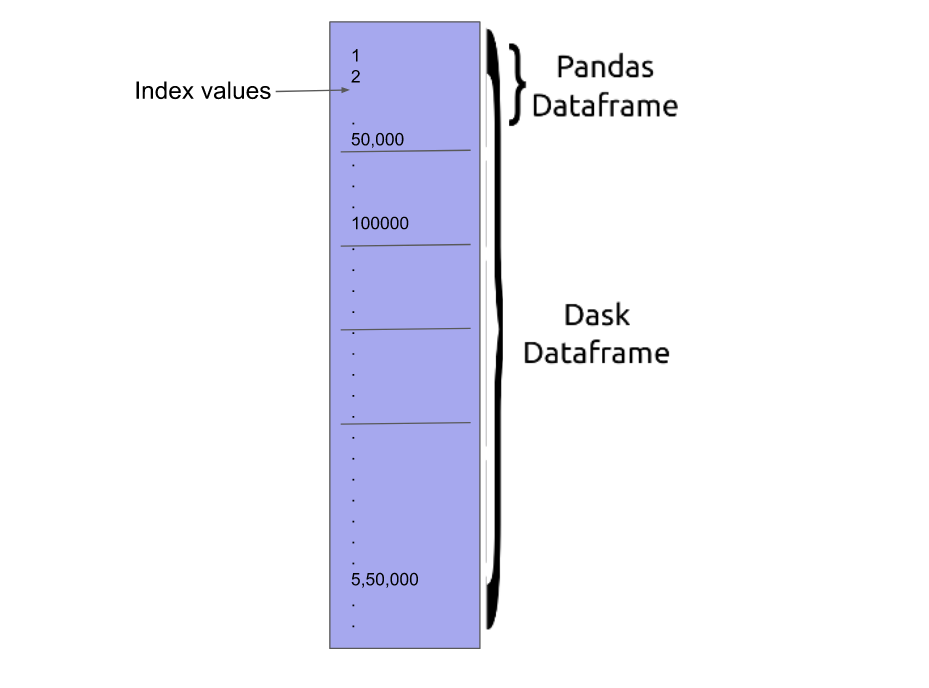The Dask Dataframe interface is very similar to Pandas, so as to ensure familiarity for pandas users. There are some differences which we shall see.

For understanding the interface, let’s start with a default dataset provided by Dask. I have used dask.datasets.timeseries() function, which can create time-series from random data.

import dask


The data_frame variable is now our dask dataframe. In padas, if you the variable, it’ll print a shortlist of contents. Let’s see what happens in Dask.

data_frame


You can see that only the structure is there, no data has been printed. It’s because Dask Dataframes are lazy and do not perform operations unless necessary. You can use the head() method to visualize data

data_frame.head()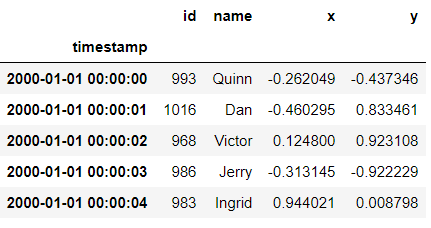Now, let’s just perform a few basic operations which are expected from pandas using dask dataframe now. One of the most standard operations is to groupby().

# Applying groupby operation
df = data_frame.groupby('name').y.std()
df


npartitions=1
float64

Name: y, dtype: float64

If you want the results then you can call compute() function as shown below.

df.compute()


name
Alice 0.575963
Bob 0.576803
Charlie 0.577633
Dan 0.578868
Edith 0.577293
Frank 0.577018
George 0.576834
Hannah 0.577177
Ingrid 0.578378
Jerry 0.577362
Kevin 0.577626
Laura 0.577829
Michael 0.576828
Norbert 0.576417
Oliver 0.576665
Patricia 0.577810
Quinn 0.578222
Ray 0.577239
Sarah 0.577831
Tim 0.578482
Ursula 0.576405
Victor 0.577622
Wendy 0.577442
Xavier 0.578316
Yvonne 0.577285
Zelda 0.576796
Name: y, dtype: float64

Sometimes the original dataframe may be larger than RAM, so you would have loaded it as Dask dataframe. After performing some operations, you might get a smaller dataframe which you would like to have in Pandas. You can easily convert a Dask dataframe into a Pandas dataframe by storing df.compute().

The compute() function turns a lazy Dask collection into its in-memory equivalent (in this case pandas dataframe). You can verify this with type() function as shown below.

# Converting dask dataframe into pandas dataframe
result_df=df.compute()
type(result_df)


pandas.core.series.Series

Another useful feature is the persist() function of dask dataframe.

So, what does persist() function do?

This function turns a lazy Dask collection into a Dask collection with the same metadata. The difference is earlier the results were not computed, it just had the information. Now, the results are fully computed or actively computing in the background.

This function is particularly useful when using distributed systems, because the results will be kept in distributed memory, rather than returned to the local process as with compute.

# Calling the persist function of dask dataframe
df = df.persist()


The majority of the normal operations have a similar syntax to theta of pandas. Just that here for actually computing results at a point, you will have to call the compute() function. Below are a few examples that demonstrate the similarity of Dask with Pandas API.

df.loc['2000-01-05']


npartitions=1
float64

Name: y, dtype: float64

Now using compute() on this materializes it.

%time
df.loc['2000-01-05'].compute()


CPU times: user 3.03 ms, sys: 0 ns, total: 3.03 ms
Wall time: 2.87 ms

Series([], Name: y, dtype: float64)

In many cases, the raw input has a lot of messy data that needs processing. The messy data is often processed and represented as a sequence of arbitrary inputs. Usually, they are processed in form of lists, dicts, sets, etc. A common problem is when they take up a lot of storage and iterating through them takes time.

Is there a way to optimize data processing at raw-level?

Dask.bag is a high-level Dask collection used as an alternative for the regular python lists, etc. The main difference is Dask Bags are lazy and distributed.

Dask Bag implements operations like map, filter, fold, and groupby on collections of generic Python objects. We prefer Dask bags because it provides the best optimization.

1. It lets you process large volumes of data in a small space, just like toolz.
2. Dask bags follow parallel computing. The data is split up, allowing multiple cores or machines to execute in parallel
3. The execution part usually consists of running many iterations. In these iterations, data is processed lazily in the case of Dask bag. It allows for smooth execution.

Because of the above points, Dask bags are often used on unstructured or semi-structured data like text data, log files, JSON records, etc.

### How to create Dask Bags?

Dask provides you different ways to create a bag from various python objects. Let’s look at each method with an example.

Method 1. Create a bag from a sequence :

You can create a dask Bag from Python sequence using the dask.bag.from_sequence() function.
The parameters are :
seq: The sequence of elements you wish to input

partition_size: An integer to denote the size of each partition

The below example shows how to create a bag from a list. After creating, you can perform a wide variety of functions on the bag. For, example, visualize() function returns a dot graph to represent the bag.

bag_1 = dask.bag.from_sequence(['Haritha', 'keerthi', 'Newton','Swetha','Sinduja'], partition_size=2)
bag_1.visualize()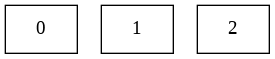Method 2. Create bag from dask Delayed objects :

You can create a dask Bag from dask Delayed objects using the dask.bag.from_delayed() function. The parameters is values. It refers to the list of dask Delayed objects you wish to input

# Creating dask delayed objects
x, y, z =[delayed(load_sequence_from_file)(fn) for fn in filenames]

# Creating a bask using from_delayed()


Method 3. Create a bag from text files:

You can create a dask Bag from a text file using the dask.bag.read_text() function. The main parameters are :

urlpath: You can pass the path of the desired text file here.

blocksize: In case the files are large, you can provide an option to cut them using this parameter

collection: It is a boolean value parameter. The function will return dask.bag if True. Otherwise will return a list of delayed values.

include_path: It is again a boolean parameter that decides
whether or not to include the path in the bag. If true, elements are tuples of (line, path). By default, it is set to False.

The below example shows how to create a bag from a textfile

b = read_text('myfiles.1.txt') # doctest: +SKIP

# Parallelize a large file by providing the number of uncompressed bytes to load into each partition

# Get file paths of the bag by setting include_path=True


Method 4. Create a Dask bag from url:

You can create a dask Bag from a URL using the dask.bag.from_url() function. You just need to input the url path, no other parameter

The below example shows how to create a bag from a url

a = dask.bag.from_url('http://raw.githubusercontent.com/dask/dask/master/README.rst',)
a.npartitions

b = dask.bag.from_url(['http://github.com', 'http://google.com'])
b.npartitions


## How to use Dask Bag for various operations?

The previous section told us the different ways of creating dask bags. Now that you are familiar with the idea, let’s see how to perform various processing operations.

For our purpose,let’s create a dask bag using the make_people() function available in dask.datasets. This function make_people() makes a Dask Bag with dictionary records of randomly generated people. To do this, it requires the library mimesis to generate records. So, you have to install that too.

!pip install mimesis
!pip install dask==1.0.0 distributed'>=1.21.6,<2.0.0' import dask import json import os # Create data/ directory os.makedirs('/content/my_data', exist_ok=True) my_bag = dask.datasets.make_people() my_bag  dask.bag The above code has successfully created a dask bag my_bag that stores information. You can also see that the number of partitions is 10. Sometimes, you may need to write the data into a disk. ### How to write the data in my_bag (of 10 partitions) into 10 JSON files and store them? In situations like these, the dask.bag.map() is pretty useful.dask. The syntax is : bag.map(func, *args, **kwargs) It is used to apply a function elementwise across one or more bags. In our case, the function to be called is json.dumps. This is responsible for writing data into JSON format files. So, provide json.dumps as input to map() function as shown below. python my_bag.map(json.dumps).to_textfiles('data/*.json')  ['data/0.json', 'data/1.json', 'data/2.json', 'data/3.json', 'data/4.json', 'data/5.json', 'data/6.json', 'data/7.json', 'data/8.json', 'data/9.json'] Yay! That was successful. Now as you might guess, dask bag is also a lazy collection. So, if you want to know or compute the actual data, you have to call the function take() or compute(). For using the take() function you need to provide input k. This k denotes that the first k elements should be taken python my_bag.take(3)  ({'address': {'address': '812 Lakeshore Cove', 'city': 'Downers Grove'}, 'age': 63, 'credit-card': {'expiration-date': '07/25', 'number': '3749 138185 40967'}, 'name': ('Jed', 'Munoz'), 'occupation': 'Clergyman', 'telephone': '+1-(656)-064-7533'}, {'address': {'address': '1067 Colby Turnpike', 'city': 'Huntington Beach'}, 'age': 62, 'credit-card': {'expiration-date': '01/17', 'number': '4391 0642 7046 4592'}, 'name': ('Emilio', 'Vega'), 'occupation': 'Sound Engineer', 'telephone': '829-959-9408'}, {'address': {'address': '572 Boardman Route', 'city': 'Lewiston'}, 'age': 28, 'credit-card': {'expiration-date': '07/17', 'number': '4521 0738 3441 8096'}, 'name': ('Lakia', 'Elliott'), 'occupation': 'Clairvoyant', 'telephone': '684-025-2843'}) You can see that first 3 data printed in above output. Now, let's move on to some processing codes. For any given data, we often perform filter operations based on certain conditions. Dask bags provides the ready-made filter() function especially for this. Let's say from my_bag collection, you want to filter out the people whose age is greater than 60. For this need to write the predicate function to check record of each age. This has to be provided as input to dask.bag.filter() function. python my_bag.filter(lambda record: record['age'] &gt; 60).take(4)


‘age’: 63,
‘credit-card’: {‘expiration-date’: ’07/25′, ‘number’: ‘3749 138185 40967’},
‘name’: (‘Jed’, ‘Munoz’),
‘occupation’: ‘Clergyman’,
‘telephone’: ‘+1-(656)-064-7533’},
‘age’: 62,
‘credit-card’: {‘expiration-date’: ’01/17′, ‘number’: ‘4391 0642 7046 4592’},
‘name’: (‘Emilio’, ‘Vega’),
‘occupation’: ‘Sound Engineer’,
‘telephone’: ‘829-959-9408’},
‘age’: 66,
‘credit-card’: {‘expiration-date’: ’11/20′, ‘number’: ‘2338 5735 7231 3240’},
‘name’: (‘Dewey’, ‘Ruiz’),
‘occupation’: ‘Green Keeper’,
‘telephone’: ‘1-445-365-1344’},
‘age’: 63,
‘credit-card’: {‘expiration-date’: ’02/20′, ‘number’: ‘4879 9327 9343 8130’},
‘name’: (‘Charley’, ‘Woods’),
‘occupation’: ‘Quarry Worker’,
‘telephone’: ‘+1-(606)-335-1595’})

The earlier discussed map() function can also be used to extract specific information. Let’s say we want to know only the occupations which people have for analysis. You can choose the occupations alone and save it in a new bag as shown below

bag_occupation=my_bag.map(lambda record: record['occupation'])
bag_occupation.take(6)


(‘Clergyman’,
‘Sound Engineer’,
‘Clairvoyant’,
‘Agent’,
‘Representative’,
‘Ornamental’)

I have printed the first 6 data stored in the processed bag above. What if you want to know many values are there in bag_occupation?

Your first go would be to do bag_occupation.count(). But, remember you won’t get any result as dask.bag is lazy. So, make sure to call compute() at the end

# computing the no of data stored
bag_occupation.count().compute()


10000

Another important function is dask.bag.groupby().
This function groups collection by key function. Below is a simple example we group even and odd numbers.

!pip install partd
iseven = lambda x: x % 2 == 0
b.groupby(iseven).compute()


[(False, [1, 3, 5, 7, 9]), (True, [0, 2, 4, 6, 8])]

It’s also possible to perform multiple data processing like filtering, mapping together in one step. This is called Chain computation. You can perform each call followed by others and finally call the compute() function. This will save memory and time. The below code is an example of Chain Computation on the my_bag collection.

result = (my_bag.filter(lambda record: record['age'] > 60)
.map(lambda record: record['occupation'])
.frequencies(sort=True)
.topk(10, key=1))
result.compute()

[(‘Councillor’, 6),
(‘Shop Keeper’, 5),
(‘Taxi Controller’, 5),
(‘Horse Riding Instructor’, 4),
(‘Press Officer’, 4),
(‘Nursing Manager’, 4),
(‘Systems Engineer’, 4),
(‘Medal Dealer’, 4),
(‘Storeman’, 4),
(‘Architect’, 4)]

Yay! we performed all processing in a single step.

### Converting Dask Bag to other forms

Many times, after processing is completed we have to convert dask bags into other forms. These other forms are generally dask dataframes, dask delayed objects, textfiles, and so on.

This section will brief you on these methods with examples.

To create Dask Dataframe from a Dask Bag, you can use dask.bag.to_dataframe() function.

Bag should contain tuples, dict records, or scalars. The index will not be particularly meaningful. Use reindex afterward if necessary.

# Converting dask bag into dask dataframe
dataframe=my_bag.to_dataframe()
dataframe.compute()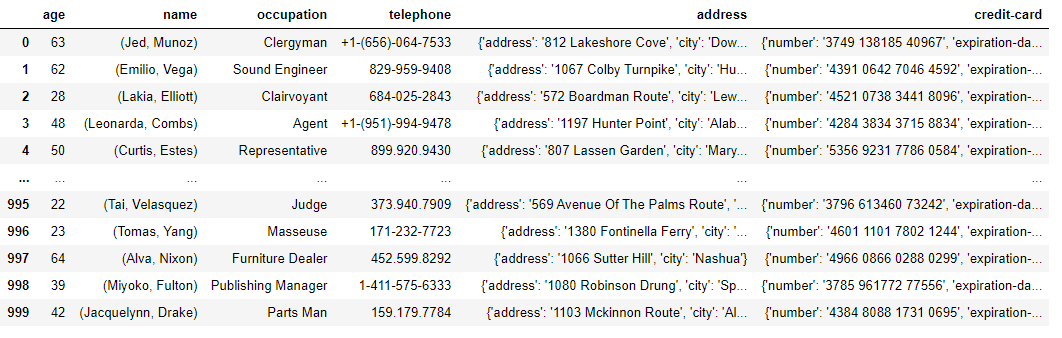2. How to create Dask.Delayed object from Dask bag

You can convert dask.bag into a list of dask.delayed objects, one per partition using the dask.bagto_delayed() function. A main parameter of this function is optimize_graph. It is a boolean parameter. If it is set to True, then the task graph will be optimized before converting it into delayed objects.

my_bag.to_delayed(True)


3. How to convert Dask bag to text files

You can write dask Bag to disk using the dask.bag.to_textfiles() function. As there are 10 partitions, 10 textfiles will be written. You have to provide the path or directory as input.

my_bag.to_textfiles('/content/textfile')


You have now learned how to create, operate and transform Dask bags. Next comes the most important concept in Dask.

## Distributed computing with Dask – Hands on Example

In this section, we shall load a csv file and perform the same task using pandas and Dask to compare performance. For this, first load Client from dask.distributed.

Dask.distributed will store the results of tasks in the distributed memory of the worker nodes. The central scheduler will track all the data on cluster. Once a result is completed, it is often erased from memory to create more space.

### What is a Dask Client?

The Client is a primary entry point for users of dask.distributed.

After we setup a cluster, we initialize a Client by pointing it to the address of a Scheduler. The Client registers itself as the default Dask scheduler, and so runs all dask collections like dask.array, dask.bag, dask.dataframe and dask.delayed.

# Import dask.distributed.Client and pandas
import pandas as pd
import time

# Initializing a client
client = Client(processes=False)
client


### Client

• Scheduler: inproc://172.28.0.2/1245/1

### Cluster

• Workers: 1
• Cores: 2
• Memory: 13.65 GB

Now, let’s do a logic / operation using pandas dataframe. Then do the same logic using dask.distibuted and compare the time taken.

First, read a csv (download from here)file into a normal pandas data frame. Clean the data and set index as per requirement. Below code prints the processed pandas data frame we have.

# Read csv file into a pandas dataframe and process it
df = df.drop('Unnamed: 0', axis=1)
df = df.set_index('itm_nb')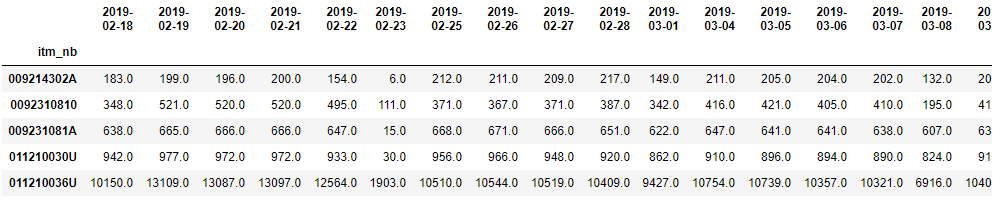dates = df.columns
for date in dates:
print(date)


Now, say we need to perform a particular function on the dataset. In the below example, for each date column, I am calculating sum of all values. We shall first execute these using pandas and record the time taken using %%time.

# A function to perform desired operation
def do_operation(df, index, date):
new_df=df[date]


Iterating through the indices of dataframe and calling the function. This is execution in pandas

%%time
# Loop through the indices and columns and call the function.
for index in df.index:
for date in dates:
do_operation(df, index, date)


CPU times: user 9.85 s, sys: 456 µs, total: 9.85 s
Wall time: 9.79 s

Observe the time taken for the above process. Now let’s see how to implement this in Dask and record the time. To reduce the time, we will use Dask client to parallelize the workload.

We had already imported and initialized a Client. Now, distribute the contents of the dataframe on which you need to do the processing using client.scatter().

To create a future, call the client.scatter() function. What will this function do?

Basically, it moves data from the local client process into the workers of the distributed scheduler.

Next, you can start looping over the indices of the dataframe. Here instead of simply calling the function, we will use client.submit() function. The client.submit() function is responsible for submitting a function application to the scheduler. To this function, you can pass the function defined, the future and other parameters.

The process is one. But, how to collect or gather the results?

We have client.gather() function for that. This function gathers futures from the distributed memory. It accepts a future, nested container of futures. The return type will match the input type. In the below example, we have passed the futures as input to this function.

%%time

# Create a futures array to store the futures returned by Dask
futures = []

# Scatter the dataframe beforehand
df_future = client.scatter(df)

for index in df.index:
for date in dates:
future = client.submit(do_operation, df_future, index, date)
# Store the returned future in futures list
futures.append(future)

# Gather the results.
_ = client.gather(futures)


Get FREE 30 DAY ACCESS  to Structured learning path for ML in Python (Beginner to Pro) –  Start as an absolute beginner and graduate over 15 courses to solve Industrial Projects like a real Data Scientist. Includes query support over chat.Reference project template for all your Data Science projects. Learn  how to load the data, get an overview of the data. Perform EDA, prepare data, build models & improve model performance.

## Similar Articles

### Logistic Regression – A Complete Tutorial With Examples in RReference project template for all your Data Science projects. Learn  how to load the data, get an overview of the data. Perform EDA, prepare data, build models & improve model performance.Course Preview

## Machine Learning A-Z™: Hands-On Python & R In Data Science

### Free Sample Videos:#### Machine Learning A-Z™: Hands-On Python & R In Data Science#### Machine Learning A-Z™: Hands-On Python & R In Data Science#### Machine Learning A-Z™: Hands-On Python & R In Data Science#### Machine Learning A-Z™: Hands-On Python & R In Data Science